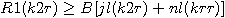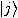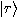## The Collected Physics Problems of William Shakespeare

Problem 1: Shall I compare thee to a summers day?
Solution:Clearly the R1 term is greater, and more temperate, under ordinary circumstances.

Problem 2: The wind does shake the darling buds of may:
Solution:This is basically a harmonic oscillator problem.
It is possible to neglect the second term provided that the wavelength is sufficiently high.

Problem 3: What light through yon window breaks? 'Tis the yeast, and Juliet is the sun.
Solution: Assume that Juliet is the sun. Therefore, J and R can have a set of joint eigenstates, to be denoted byandas seen by equation 4.120. Note that (6.55) has the structure of a one-dimensional equation in r whose solutions give the energy levels of the system for which the wave function is given by equation 6.57. Therefore the light consists of quantized particles. But Juliet must pay to replace the window.
 This English literature-related article is incomplete. You can help by sending in more physics-related Shakespeare jokes.

Back